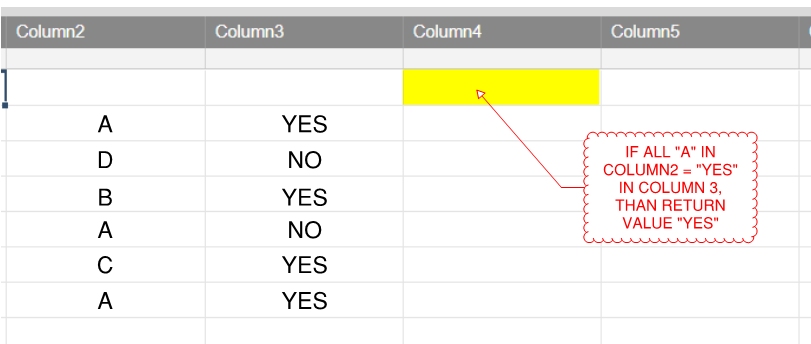# IF WITH 2 CRITERIA

I'm looking to make a formula that will do the following:

search all instances in Column2 for "A" IF subsequent Column3  has a value of "YES"....if all "A"s = "YES" than return a value of "YES" otherwise "NO"

I included an image that hopefully clarifies this a little more.

in the image I show 3 "A"s in Column2, 2=YES and 1=NO in Column3...so therefore the formula would return a value of "NO"• Hi,

you could try to check if the count of "NO" is >0 for the range where Column2="A". This would look like :

"=IF(COUNTIF(COLLECT(COLUMN3:COLUMN3,COLUMN2:COLUMN2,"A"),"NO")>0,"YES","NO")"

Could this help?

Cheers,

Paul.

## Help Article Resources

Want to practice working with formulas directly in Smartsheet?

Check out the Formula Handbook template!